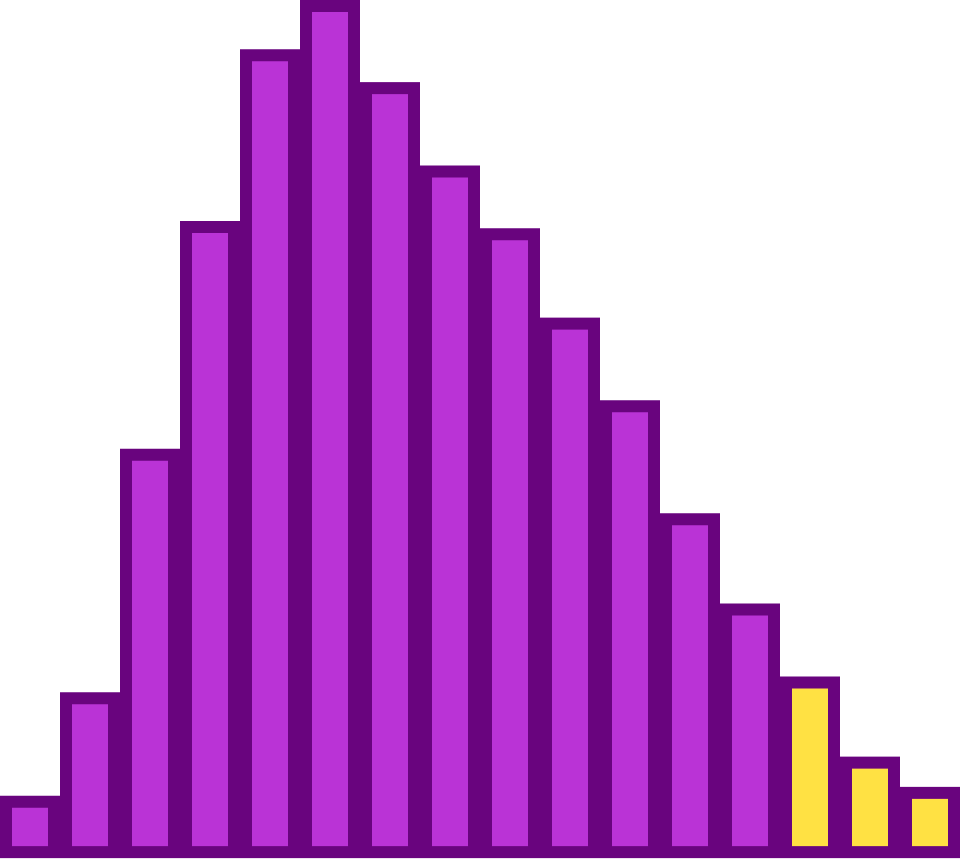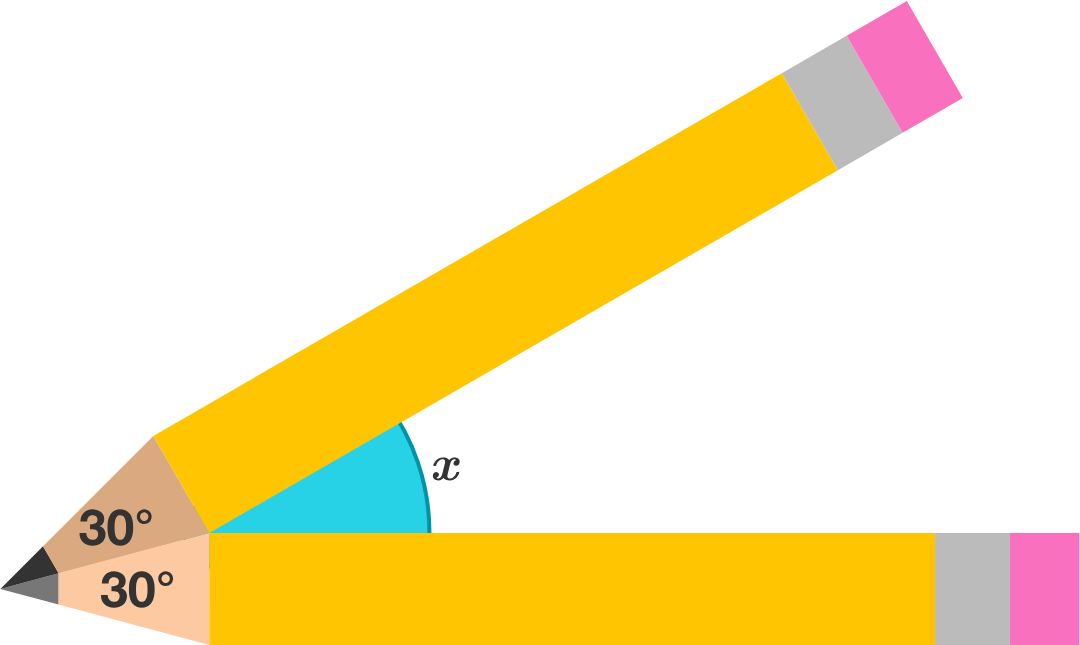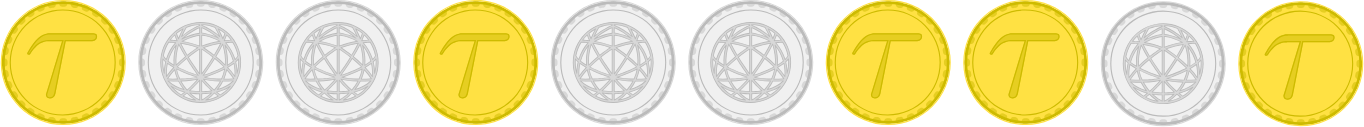# Core TopicsContest Math II explores the topics and techniques needed to excel in mathematical problem-solving, especially for exams at the level of the American Mathematics Competition (AMC).

Through carefully crafted problems and guided explanations, you'll improve your skills in the core topics: Algebra, Geometry, Number Theory, and Discrete Math. This quiz introduces problems from all of these topics.

# Core Topics

Algebra

$\large x+\frac{1}{x} = 5, \qquad x^2 + \frac{1}{x^2} = \, ?$

# Core Topics

Geometry

If the angle of each pencil tip in diagram is $30 ^ \circ,$ then what is $x?$In this flat diagram, each identically shaped pencil is formed by a rectangle attached to an isosceles triangle.

# Core Topics

Number Theory

What is the greatest common divisor of $2017!$ and $2017!+1?$

# Core Topics

Discrete Math

Each coin shown has a gold side and a silver side. For the above arrangement, is it possible to successively flip over pairs of adjacent coins so that all of the coins have their gold side facing up?

"Flip" indicates switching from gold to silver or back again, not a random flip like in probability. "Pairs of adjacent coins" means only two coins next to each other may be flipped at a time.

# Core Topics

In the previous problem, it is possible to "play" with the coins and determine that it seems impossible to get all gold.

However, one of the skills which you'll develop in Contest Math II is the ability to generalize from patterns with small cases.

In particular, note that flipping pairs of coins does not change the parity (even/odd) of the total number of gold coins. In this case, since there was initially an odd number of gold coins, it is impossible to ever have four gold coins.

In the next problem, you'll apply this observation to a more general case.

# Core TopicsEach coin shown has a gold side and a silver side. Is it possible to flip over pairs of adjacent coins so that all of the coins have their gold side facing up?

"Flip" indicates switching from gold to silver or back again, not a random flip like in probability. "Pairs of adjacent coins" means only two coins next to each other may be flipped at a time.

×

Problem Loading...

Note Loading...

Set Loading...